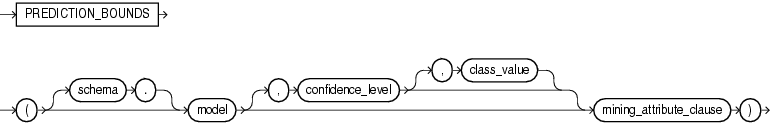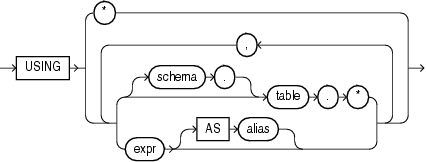# PREDICTION_BOUNDS

SyntaxDescription of the illustration prediction_bounds.gif

mining_attribute_clause::=Description of the illustration mining_attribute_clause.gif

Purpose

The `PREDICTION_BOUNDS` function is for use only with generalized linear models. It returns an object with two `NUMBER` fields `LOWER` and `UPPER`. For a regression mining function, the bounds apply to value of the prediction. For a classification mining function, the bounds apply to the probability value. If the GLM was built using ridge regression, or if the covariance matrix is found to be singular during the build, then this function returns `NULL` for both fields.

• For `confidence_level`, specify a number in the range (0,1). If you omit this clause, then the default value is 0.95.

• The `class_value` argument is valid for classification models but not for regression models. By default, the function returns the bounds for the prediction with the highest probability. You can use the `class_value` argument to filter out the bounds value specific to a target value.

You can specify `class_value` while leaving `confidence_level` at its default by specifying `NULL` for `confidence_level`.

• The `mining_attribute_clause` has the same behavior for `PREDICTION_BOUNDS` that it has for `PREDICTION`. Refer to mining_attribute_clause.

Example

The following hypothetical example returns the distribution of customers whose ages are predicted to be between 25 and 45 years with 98% confidence. The example is presented here to illustrate the syntactic use of the function. General information on data mining demo files is available in Oracle Data Mining Administrator's Guide.

```SELECT count(cust_id) cust_count, cust_marital_status
FROM (SELECT cust_id, cust_marital_status
FROM mining_data_apply_v
WHERE PREDICTION_BOUNDS(glmr_sh_regr_sample,0.98 USING *).LOWER > 24 AND
PREDICTION_BOUNDS(glmr_sh_regr_sample,0.98 USING *).UPPER < 46)
GROUP BY cust_marital_status;

CUST_COUNT CUST_MARITAL_STATUS
-------------- --------------------
46 NeverM
7 Mabsent
5 Separ.
35 Divorc.
72 Married
```